• +91 9971497814
• info@interviewmaterial.com

# Chapter 9 Sequences and Series Ex-9.4 Interview Questions Answers

### Related Subjects

Question 1 :

Find the sum to n terms of each of the series in Exercises 1 to 7.

1 × 2 + 2 × 3 + 3 × 4 + 4 × 5 + …

Given series is 1 × 2+ 2 × 3 + 3 × 4 + 4 × 5 + …

It’s seen that,

nth term, an = n ( n +1)

Then, the sum of nterms of the series can be expressed as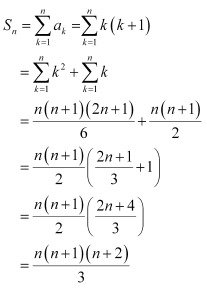Question 2 :

1 × 2 × 3 + 2 × 3 × 4 + 3 × 4 × 5 + …

Given series is 1 × 2× 3 + 2 × 3 × 4 + 3 × 4 × 5 + …

It’s seen that,

nth term, an = n ( n +1) ( n + 2)

= (n2 + n)(n + 2)

= n+3n+ 2n

Then, the sum of nterms of the series can be expressed as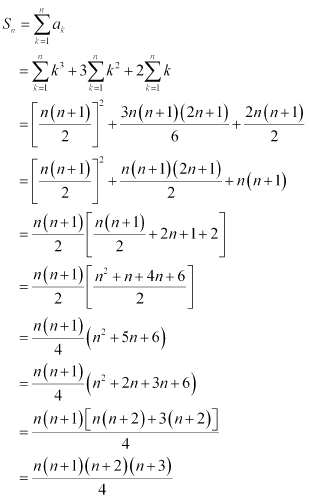Question 3 : 3 × 12 + 5 × 22 +7 × 32 + …

Given series is 3 ×12 +5 × 22 + 7 × 32 + …

It’s seen that,

nth term, an = ( 2n +1) n2 = 2n3 + n2

Then, the sum of nterms of the series can be expressed as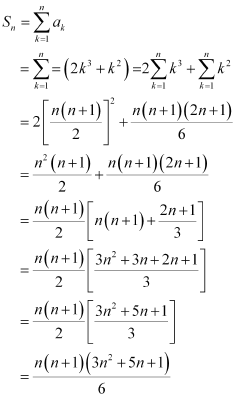Question 4 :

Find the sum to n terms of the series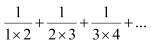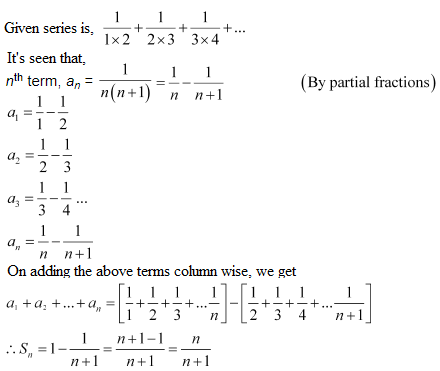Question 5 :

Find the sum to n terms of the series 52 +62 + 72 + … + 202

Given series is 52 +62 + 72 + … + 202

It’s seen that,

nth term, an = ( n +4)2 = n2 + 8n + 16

Then, the sum of nterms of the series can be expressed as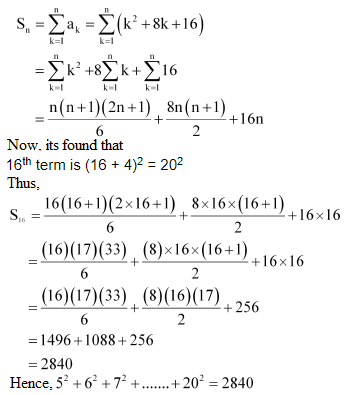Question 6 : Find the sum to n terms ofthe series 3 × 8 + 6 × 11 + 9 × 14 +…

Given series is 3 × 8+ 6 × 11 + 9 × 14 + …

It’s found out that,

a= (nth term of 3, 6, 9…) × (nth term of 8, 11, 14, …)

= (3n) (3n +5)

= 9n2 +15n

Then, the sum of nterms of the series can be expressed as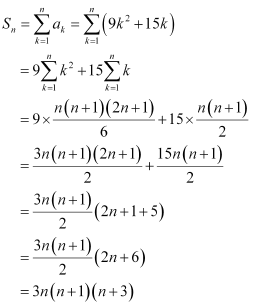Question 7 :

Find the sum to n terms of the series 12 +(12 + 22) + (12 + 22 +32) + …

Given series is 12 +(12 + 22) + (12 + 2+32 ) + …

Finding the nth term,we have

an = (12 + 22 +32 +…….+ n2)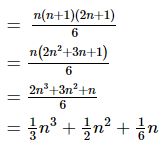Now, the sum of nterms of the series can be expressed as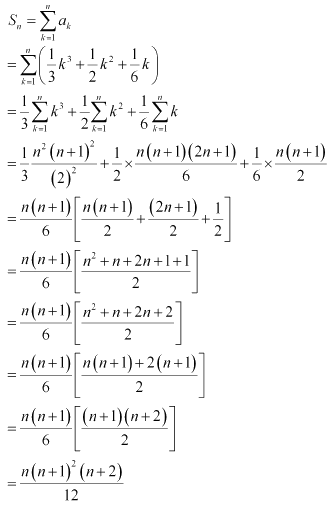Question 8 :

Find the sum to n terms of the series whose nth termis given by n (n + 1) (n + 4).

Given,

an = n (n + 1)(n + 4) = n(n+ 5n +4) = n3 + 5n2 + 4n

Now, the sum of nterms of the series can be expressed as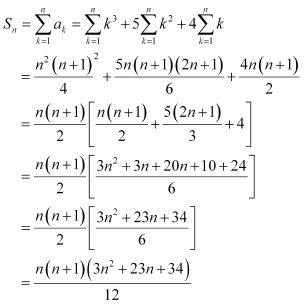Question 9 : Find the sum to n terms ofthe series whose nth terms is given by n2 +2n

Given,

nth termof the series as:

an = n2 + 2n

Then, the sum of nterms of the series can be expressed as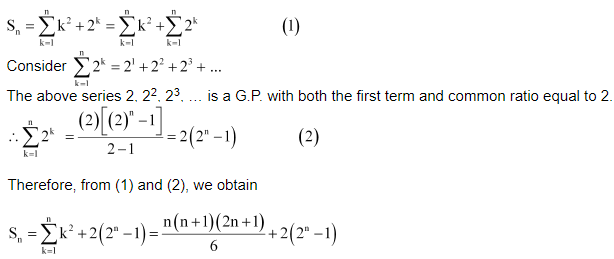Question 10 :

Find the sum to n terms of the series whose nth termsis given by (2n – 1)2

Given,

nth termof the series as:

an = (2n – 1)2 =4n2 – 4n + 1

Then, the sum of nterms of the series can be expressed as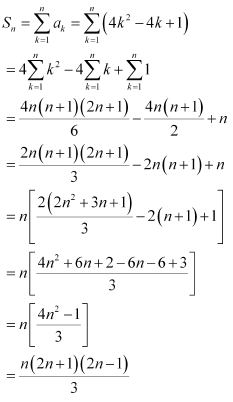Todays Deals### Chapter 9 Sequences and Series Ex-9.4 Contributorskrishan

Name:
Email:

# Latest News# 9000 interview questions in different categories